Open in App
Not now

# Engineering Mathematics – Partial Derivatives

• Difficulty Level : Basic
• Last Updated : 23 Nov, 2022

A function is like a machine that takes some input and gives a single output. For example, y = f(x) is a function in ‘x’. Here, we say ‘x’ is the independent variable and ‘y’ is the dependent variable as the value of ‘y’ depends on ‘x’.

Some examples of functions are:

1. f(x) = x2 + 3 is an algebraic function.
2. e is the exponential function.
3. sin(x), cos(x), tan(x),…etc. are all trigonometric functions.

Now, all these functions are functions of a single variable, i.e. there is only one independent variable.

To understand the concept of partial derivative, we must first look at what a function in two variables means.
Consider a function of the form z = f(x,y) where ‘x’ and ‘y’ are the independent variables and ‘z’ is the dependent variable. This function is called a function in two variables. Similarly, functions of several variables (i.e. with more than 2 independent variables) can also be defined.

Some examples of multivariable functions or functions of several variables are:

1. f(x,y) = x2 +y

2. f(x,y,z) = x-3y+4z

Let us visualize this concept through graph. First we consider a single variable function f(x) = x2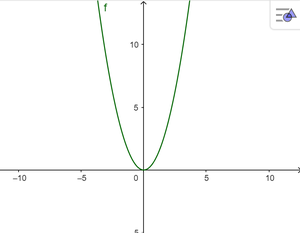Graph of f(x) = x^2

Unlike functions of a single variable, we cannot visualize multivariable functions as a 2-D graph. For this, we plot it on the 3-D plane. For example, consider the graph of f(x,y) = x2+y2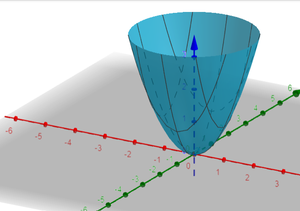Graph for f(x,y) = x^2 + y^2

For functions of several variables we define the limit as follows: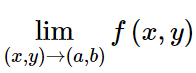This means, finding the limit of f(x) as ‘x’ approaches ‘a’ and ‘y’ approaches ‘b’.

Similarly, the definitions of continuity and differentiability can be extended from definitions for single variable functions.

Recall that the derivative of a function of single variable y=f(x) is defined as : f'(x) = `For a function z = f(x,y) of two variables, we define the derivative as :This means calculating the derivative of function ‘z’ with respect to ‘x’ by keeping ‘y’ constant. Similarly, we can calculate the derivative of ‘z’ with respect to ‘y’ by keeping ‘x’ as constant as### Geometrical Interpretation of the partial derivative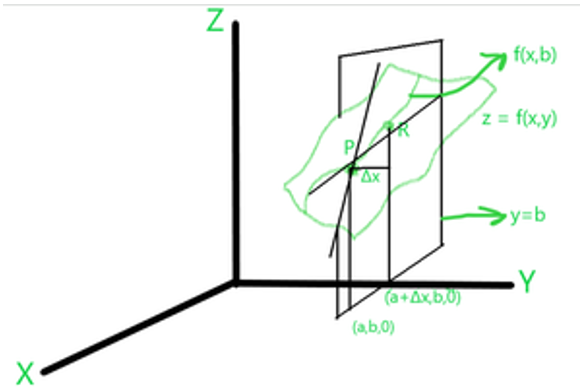As we know, for single variable functions, the derivative is computed as the slope of the tangent passing through the curve. Similarly, we can understand the geometric interpretation of a partial derivative of a multivariable function.

Consider a function of two variables, z = f(x,y) on the 3-D plane and let a plane y=b pass through the curve f(x,y).

Now, we draw another curve f(x,b) lying on z that is perpendicular to the plane y=b. Consider two arbitrary points P,R on this curve and draw the secant passing through these points.

The slope of this secant is calculated using the first principles as follows :As the two points move closer to each other, the difference Δx approaches 0 and we calculate this in the form of the limit :This limit is the partial derivative of ‘z’ with respect to ‘x’ by treating ‘y’ as constant i.e.### Computing partial derivatives of a given function.

Steps to calculate partial derivative of a given function :

1. Consider z = f(x,y).
2. Compute partial derivative with respect to ‘x’ i.e.by considering ‘y’ as constant and differentiate the function with respect to ‘x’.
3. Compute partial derivative with respect to ‘y’ i.e.by considering ‘x’ as constant and differentiate the function with respect to ‘y’.

ExampleHere, for the given function, we calculate the two partial derivatives as follows :

Case 1: Differentiating with respect to ‘x’ by treating ‘y’ as constant i.e.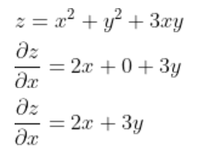Differentiating ‘z’ wrt ‘x’ by treating ‘y’ constant

Case 2: Differentiating with respect to ‘y’ by treating ‘x’ as constant i.e.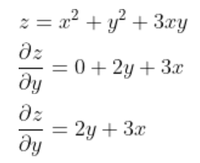Differentiating ‘z’ wrt ‘y’ by treating ‘x’ constant

### Second-Order Partial Derivatives

Similar to the computation of second-order derivatives for functions of single variables, we can compute the same for functions of several variables.

For an example we consider the same function.

Case 1: We differentiateagain with respect to ‘x’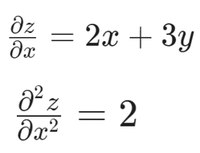Case 2: We differentiateagain with respect to ‘y’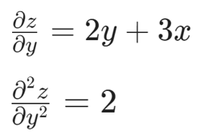Case 3: We differentiateagain with respect to ‘y’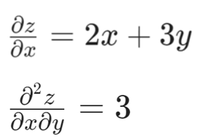Case 4: We differentiateagain with respect to ‘x’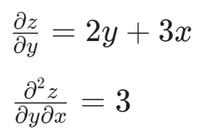My Personal Notes arrow_drop_up